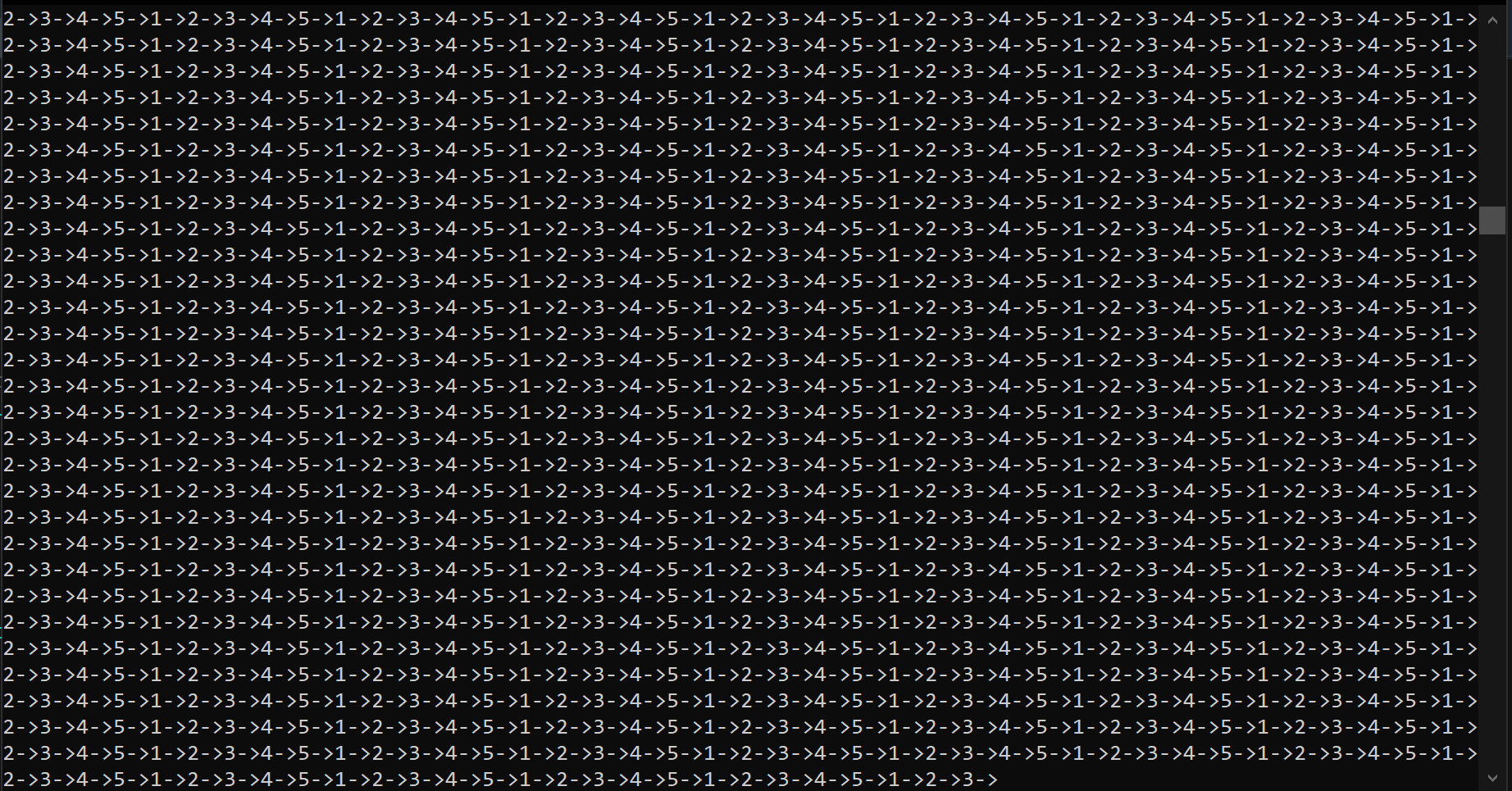Created June 22, 2021

To learn the basics of a linked lists you can check out my other posts here.

So let's get started!

So what exactly do we mean by a linked list? A circular linked list is a normal linked list but with its head and last element connected.## 1. Insertion

The code is very similar to a normal linked list. The only difference is that whenever we insert an element we'll search the last element by traversing to the element that has its next pointed towards the head node instead of null.

This is how the insertion process looks like in C:

``````NODE *insertnode(NODE *head, int data){
NODE *newnode = createnode(data);
}
else{
//traverse to the last element and insert the newnode there
temp = temp->next;
}//temp reaches the last element;
temp->next = newnode; //add the last element after the last element in the ciruclar linked list
newnode->next=head;  //after adding the newnode point its next to the head node so as to make it circular.
}
}
``````

As you can see, here we have traversed to the node that has its next pointed to the head node.

## Traversal

No element points at null as that would mean the end of the list and circles do not have any end or beginning. So, the name is pretty much self-explanatory. Let's look at an example! What if we try to traverse through this list until we reach the element which points at null, just like how we do in a normal linked list. Here's the code snippet for that:

``````void traverse(NODE *head){
while(temp!=NULL){
printf("%d->", temp->data);
temp = temp->next;
}
}
``````

If you try to run this code for a circular linked list it will probably result in something like this -That happened because there's no end to this list! So, all you have to do is change the condition for the while loop in the above code. It would then look something like this:

``````void traverse(NODE *head){
printf("%d->", temp->data);
temp = temp->next;
}printf("%d->", temp->data);
printf("\n");
}
``````

Now let's see the deletion process.

## Deletion

The deletion part is also very similar to a normal linked list. The only difference would be deletion of the last element of the list. If you want to delete the last element, then make the node before that element to point at the head node and free the target node.

``````void deletenodelast(NODE *head){
//traverse to the last node;
temp = temp->next;
}//temp reaches the second last node;
//set temp1 equal to the target node;
temp1 = temp->next;
//set the next of the second last node to the head node;